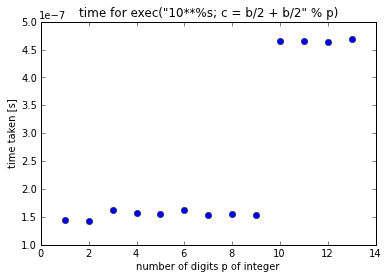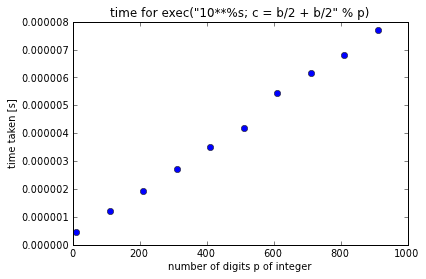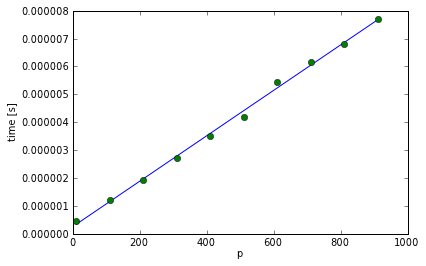# Performance of Python's long data type

Python 2.x has two integer datatypes: the int and the long. (In Python 3.x, the distinction disappears.) The int data type corresponds to the 'normal' integer data type that the CPU supports. The range of that data type can be obtained from the maxint attribute in the system module:

In :
import sys
a = sys.maxint
print(a)

2147483647


The object bound to the name a is the largest integer of type int in Python:

In :
type(a)

Out:
int

Operations using integers below this limit sys.maxint are carried out using the hardware's computational capabilities. When the range is exceeded, Python (silently) changes the type from int to long:

In :
type(a + 1)

Out:
long
In :
a + 1

Out:
2147483648L

Note the L at the end of the number: this shows that the integer displayed is of type long and not of type int.

Large integer numbers occur every now and then. One example is that of working out propabilities for events using fractions of possible combinations, see for example the entry on the likelihood of two people having the same birthday in a group of $r$ people at http://rebrained.com/?p=317 (you may need to click on 'answer' on that page to see the full story). An expression like $365^r$ exceeds sys.maxint for the system used here for $r \ge 4$.

In principle, there is no upper limit to the size of the integers of type long. However, as the computation is now done at the software level (where any mathematical operations with objects of type long have to be reduced to those that the CPU can carry out), there is a performance penalty in comparison to using the (hardware-supported) int objects.

# How much slower is long in comparison to int?¶

In :
import timeit
N = 10 ** 5
min(timeit.repeat('%d / 2 + %d / 2' % (a, a), number=N, repeat=10)) / N

Out:
2.0206928253173827e-07

So the operation of taking an integer object a, and dividing it by 2, and adding it to a / 2, takes about 2e-7 = 0.15 micro seconds.

How long does it take to do the same operation a / 2 + a / 2 with an a of type long?

In :
b = sys.maxint + 1
type(b)

Out:
long
In :
min(timeit.repeat('%d / 2 + %d / 2' % (b, b), number=N, repeat=10)) / N

Out:
4.984903335571289e-07

It takes about about 3 times as long as for the int. That is actually not so bad.

The timeit module is a convenient tool to time small pieces of Python code. It carries out the operation many times (N times) and thus this will average over CPU load fluctuations on the system and give better performance data than a single execution of the statement. It also repeats the whole measurement (here 10 times), and returns a list with 10 numbers, each corresponding to the time taken for one measurement. Following the recommended procedure, we take the minimum time of these repeated measurements as the best number for execution time.

It might be interesting to look at the time it takes for the same integer operation as a function of the size of the number. In particular, let us do the same operation for numbers $x = 1, 10, 100, 1000, \ldots, 10^p$ which we label by the number of digits $p$ through $x = 10^p$.

In :
import pylab
def measure_long_calc(powers):
ts = []
N = 100000
for p in powers:
cmd = 'b=10 ** %s; c = b / 2 + b / 2;' % (p)
t = min(timeit.repeat(cmd, number=N, repeat=10))/N
ts.append(t)
print("t=%10.3e p=%s" % (t,p))
pylab.plot(powers,ts,'o')
pylab.xlabel('number of digits p of integer')
pylab.ylabel('time taken [s]')
pylab.title('time for exec("10**%s; c = b/2 + b/2" % p)')
pylab.savefig('plot.png')
pylab.savefig('plot.pdf')
return powers, ts

In :
ps, ts = measure_long_calc(range(1,14))

t= 1.436e-07 p=1
t= 1.435e-07 p=2
t= 1.626e-07 p=3
t= 1.577e-07 p=4
t= 1.553e-07 p=5
t= 1.618e-07 p=6
t= 1.537e-07 p=7
t= 1.544e-07 p=8
t= 1.535e-07 p=9
t= 4.652e-07 p=10
t= 4.644e-07 p=11
t= 4.639e-07 p=12
t= 4.690e-07 p=13We can see that calculation time is approximately constant for up to 9 digits. This is the range of numbers up to 1,000,000,000 which is below sys.maxint as shown above, and thus the operations are carried out in the hardware.

For 10 and more digits, the numbers $\ge 10^{10}$ are emulated in software, and thus execution time increases significantly. As seen above, the step is approximately from 0.15 micro seconds to 0.6 microseconds, i.e. roughly a factor 3 to 4.

As the long data type has no limit on the number of digits in a long integer number, one may ask how the computation time changes as a function of the length of a long number. Repeating the calculation above for a wider set of values $p$, now ranging up to 900 digits, results in the following data:

In :
ps, ts = measure_long_calc(range(11,1000,100))

t= 4.715e-07 p=11
t= 1.198e-06 p=111
t= 1.932e-06 p=211
t= 2.707e-06 p=311
t= 3.489e-06 p=411
t= 4.197e-06 p=511
t= 5.427e-06 p=611
t= 6.150e-06 p=711
t= 6.818e-06 p=811
t= 7.699e-06 p=911The execution time increases linearly with the number of digits.

We can fit a linear polynomial (i.e. $f(x) = c_0 + c_1 x$) through the plotted data to find

In :
from numpy import polyfit
c1, c0 = polyfit(ps, ts, 1)
y = c0+c1*np.array(ps)
plot(ps, y,'-')
plot(ps,ts,'o')
xlabel('p')
ylabel('time [s]')
print("Coefficient c1=%.3g (and c0=%g)." % (c1, c0))

Coefficient c1=8.14e-09 (and c0=2.55216e-07).Following this model and fit over a large range of digits p, it takes $c_1 \approx 8\mathrm{ns} = 0.08 \mu\mathrm{s}$ per digit in the variable of type long for the calculations used for these timings, plus an offset of $c_0$ seconds.

The time for the hardware based calculation for up to 11 digits was $\approx 0.15 \mu\mathrm{s}$. A quick consistence check: according to the fitted linear model, 11 digits software based calculation would take $11c_1 + c_0$, i.e.

In :
c1 * 11 + c0

Out:
3.4477879784323773e-07

The time we had found above for the smallest long calculation was about 4.5e-07.

Finally, the system used for these timings is a MacBook Air, 11 inch, Mid 2012, 1.7 GHz Intel Core i5, Memory 8 GB 1600 MHz DDR3, OS X 10.8.2, Python 2.7 (Enthought Distribution) with Ipython 0.13.1.